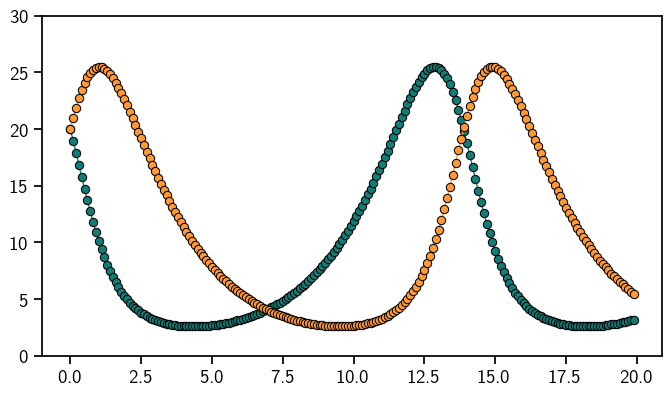# Tutorials¶

Learn how to use ProbNum and get to know its features. You can interactively try out thedirectly in the browser or by downloading the notebooks from the GitHub repository.

## Getting Started¶## Features of ProbNum¶

### Linear Solvers¶

Solving linear systems is arguably one of the most fundamental computations in statistics, machine learning and numerics. For example, linear systems arise when inferring parameters in statistical models or during model training. ProbNum provides a family of linear solvers, which infer either the inverse system matrix or the solution directly, while quantifying their uncertainty.### Ordinary Differential Equation Solvers¶

The behaviour of complex systems which evolve over time is often described via the use of ordinary differential equations. ProbNum provides a set of methods to solve ordinary differential equations based on filtering approaches which quantify the uncertainty introduced by discretization.### Bayesian Filtering and Smoothing¶

Bayesian filtering and smoothing provides a framework for efficient inference in state space models. For non-linear state space components, ProbNum provides linearization techniques that enables Gaussian filtering and smoothing in more complex dynamical systems.### Linear Operators¶

Linear algebra is essential to virtually all of scientific computation. ProbNum implements (finite-dimensional) linear operators in a memory-efficient manner with support for lazy arithmetic.### Probability¶

Random variables are the main objects in ProbNum. They represent uncertainty about a numerical quantity via their distribution. A probabilistic numerical method takes random variables as inputs and also outputs random variables.